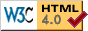# TPC Probability Statements

This is a message I posted to ne.weather on August 27, 1996.

I have a number of criticisms of the TPC's (Tropical Prediction Center, formerly National Hurricane Center) probability charts for tropical cyclones. I have finally decided to mention these on the group. I hope that someone from TPC or is otherwise knowledgable will respond.

The main problem is that, mathematically, they just don't make sense. There are two specific problems I see:

The first problem is that when the forecast positions are given, the probabilities don't make sense with the forecast positions. As an example, I will use

```TROPICAL DEPRESSION SEVEN PROBABILITIES NUMBER   1
NATIONAL WEATHER SERVICE MIAMI FL
5 PM AST TUE AUG 27 1996
```

The three items given in this statement are all forecast positions:

```LOCATION           A  B  C  D  E   LOCATION           A  B  C  D  E

10.5N  36.0W      45  X  X  X 45   10.5N  40.0W       X 13  6  2 21
10.5N  38.0W       8 19  X  1 28

COLUMN DEFINITION   PROBABILITIES IN PERCENT
A IS PROBABILITY FROM NOW TO  2PM WED
B FROM  2PM WED TO  2AM THU
C FROM  2AM THU TO  2PM THU
D FROM  2PM THU TO  2PM FRI
E IS TOTAL PROBABILITY FROM NOW TO  2PM FRI
X MEANS LESS THAN ONE PERCENT
```

The first position given, 10.5°N 36.0°W, is the forecast position for 28/1800Z, or 2PM WED. The probability of the system passing within 65 miles before the forecast time is 45%, yet the probability of passing within 65 miles after the forecast time is 0%. One would expect these probabilities to be about equal. The same is true for the second position as well (B and C should be about equal).

This is very consistent with my observations of other statements.

This could be explained by the fact that it is the probability of entering the region within 65 miles of that location during the probability period, but this does not seem to account for as much difference as exists.

The second concern I have is that probabilities are added when computing column E in these statements. Probabilities are rarely additive, and they are not in this case (only when only one of A, B, C, and D is possible). However, the probability of a hurricane passing within 65 miles of a location in one time period is correlated with the probability of it passing within 65 miles of the location in another period, mainly because systems can easily stay within the area, and also because they can enter twice. Eight hours, or even much longer in weak steering currents, is a reasonable amount of time for a system to travel 100-130 miles. Thus, E should be lower still then that obtained from the standard mathematical formula I give below, where probabilities are assumed to be uncorrelated. (Note that there would be less correlation (meaning the formula I give would be closer) or more likely a negative correlation (meaning E would be between TPC's formula and the one I give), if the probabilities were for entering the 65 mile range, since systems are less likely to enter twice.)

Here is a line from a DOLLY statement last week:

```22.0N  99.5W      18 10  1  X 29
```

If the time periods were uncorrelated, column E would be:

```E=100%-(probability it is in no other columns)
=100%-((100%-A)(100%-B)(100%-C)(100%-D))          <-- THE FORMULA
```

which in this case would be 27%, not much of a difference.

However, when the probabilities are higher, the difference is greater (especially when they add to more than 100%!)

Two more hypothetical examples, though I have seen things like them:

```A  B  C  D  E
43 22 17 2  84
```

E should be under 63%. (63% if uncorrelated)

```A  B  C  D  E
99 12 X  X  99
```

Well, you can't add here. This is correct by all methods, though if TPC were consistent, they would give 111%!

In addition, they never go above 99 (even if it is already within 65 miles!) to 100, which they should do in these cases.

These observations lead me towards the conclusion that there is certainly no formula for computing these probabilities, and probably no standard way of doing so. Rather, the forecasters just sit down and write the probabilities. The statement, at first glance, seems deceptively calculated and formula based (as TRANTECH's is.)

TRANTECH's strike charts, being in a different, and, IMHO, far superior, format, do not suffer from these problems.

The only use of the TPC statements seems to be in gauging the uncertainty of the forecasters.

David Baron(Back to Views, David Baron)